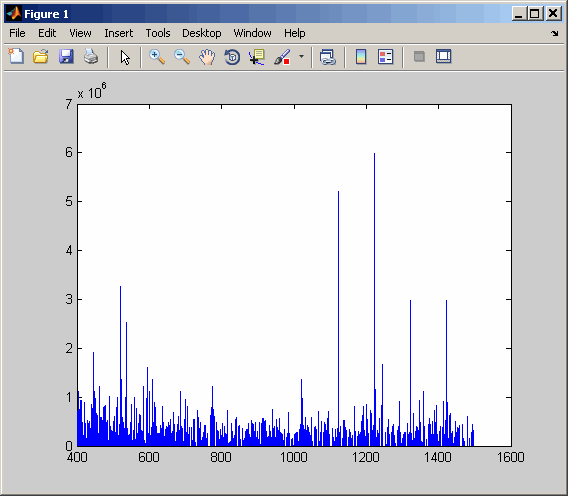## Syntax

``mzXMLStruct = mzxmlread(myFile)``
``mzXMLStruct = mzxmlread(myFile,Name,Value)``

## Description

example

````mzXMLStruct = mzxmlread(myFile)` returns a MATLAB® structure, `mzXMLStruct`, from an mzXML file, `myFile`.```

example

````mzXMLStruct = mzxmlread(myFile,Name,Value)` reads an mzXML file, `myFile`, and then returns a MATLAB structure, `mzXMLStruct`, using additional options specified by one or more `Name,Value ` pair arguments.```

## Examples

collapse all

In this example, the file `results_1.mzxml` is not provided. You can find sample mzXML files at:

Read an mzXML file into a MATLAB structure.

`out = mzxmlread('results_1.mzxml')`
```out = scan: [2000x1 struct] mzXML: [1x1 struct] index: [1x1 struct] ```

View the first scan in the mzXML file by creating separate variables containing the mass-to-charge ratio (`mz_ratio`) and intensity (`Y`) values respectively. Then plot these values.

```mz_ratio = out.scan(1).peaks.mz(1:2:end); Y = out.scan(1).peaks.mz(2:2:end); stem(mz_ratio,Y,'marker','none')```In this example, the file `results_2.mzxml` is not provided. You can find sample mzXML files at:

Read an mzXML file into a MATLAB structure, extracting a scan at index 1000.

`out1 = mzxmlread('results_2.mzxml','ScanIndices',1000)`
```out1 = scan: [1x1 struct] mzXML: [1x1 struct] index: [1x1 struct]```

Read an mzXML file into a MATLAB structure, extracting multiple scans at indices 1000, 1500, and 2000.

`out2 = mzxmlread('results_2.mzxml','ScanIndices',[1000 1500 2000])`
```out2 = scan: [3x1 struct] mzXML: [1x1 struct] index: [1x1 struct]```

Read an mzXML file into a MATLAB structure, extracting a range of scans from indices 1000 to 2000.

`out3 = mzxmlread('results_2.mzxml','ScanIndices',[1000:2000])`
```out3 = scan: [1001x1 struct] mzXML: [1x1 struct] index: [1x1 struct] ```

## Input Arguments

collapse all

Input file, specified as a character vector or string containing an mzXML file name. The file must conform to the mzXML 2.1 or earlier specifications. You can read the mzXML 2.1 specification here:

### Name-Value Arguments

Specify optional pairs of arguments as `Name1=Value1,...,NameN=ValueN`, where `Name` is the argument name and `Value` is the corresponding value. Name-value arguments must appear after other arguments, but the order of the pairs does not matter.

Before R2021a, use commas to separate each name and value, and enclose `Name` in quotes.

Example: `'TimeRange',[5.0 10.0],'Verbose',true`

Spectra levels, specified as a positive integer or vector of integers indicating which scans to extract scans from `myFile`. By default, `mzxmlread` reads all spectra levels.

For summary information about the levels of spectra in an mzXML file, use the `mzxmlinfo` function.

If you are using the `'Levels'` name-value pair argument, then you cannot use `'ScanIndices'`.

Example: `'Levels',5`

Range of time, specified as a two-element numeric array, such as `[Start End]` indicating which scans to extract from `myFile`. The `Start` and `End` scalar values must be between the `startTime` and `endTime` attributes of the `msRun` element in `myFile`. The `Start` scalar value must be less than `End`. By default, `mzxmlread` reads all scans.

For summary information about the time ranges in an mzXML file, use the `mzxmlinfo` function.

If you are using `'TimeRange'` name-value pair argument, then you cannot use `'ScanIndices'`.

Example: `'TimeRange',[5.1 10.2]`

Scan indices, specified as a positive integer or vector of positive integers indicating which scans to extract from `myFile`. Use an integer to specify a single scan, or a vector of integers to specify multiple scans. By default, `mzxmlread` reads all scans.

For summary information about the time ranges in an mzXML file, use the `mzxmlinfo` function.

If you are using the `'ScanIndices'` name-value pair argument, then you cannot use `'Levels'` or `'TimeRange'`.

Example: `'ScanIndices',7000`

Verbose mode, specified as `true` (`1`), or `false` (`0`). When `'Verbose'` is set to `true`, `mzxmlread` displays the progress while reading `myFile`.

Example: `'Verbose',true`

## Output Arguments

collapse all

Structure from an mzXML file, returned as a MATLAB structure. `mzXMLStruct` has the following fields:

FieldDescription
`scan`Structure array containing the data pertaining to each individual scan, such as mass spectrometry level, total ion current, polarity, precursor mass (when it applies), and the spectrum data.
`index`Structure containing indices to the positions of scan elements in the XML document.
`mzXML`

Structure containing all of the following:

• Information in the root element of the mzXML schema, such as instrument details, experiment details, and preprocessing methods

• URLs pointing to schemas for each scan

• Indexing approach

• Digital signature calculated for the current instance of the document

## Tips

LC/MS data analysis requires extended amounts of memory from the operating system.

## Version History

Introduced in R2006b